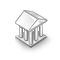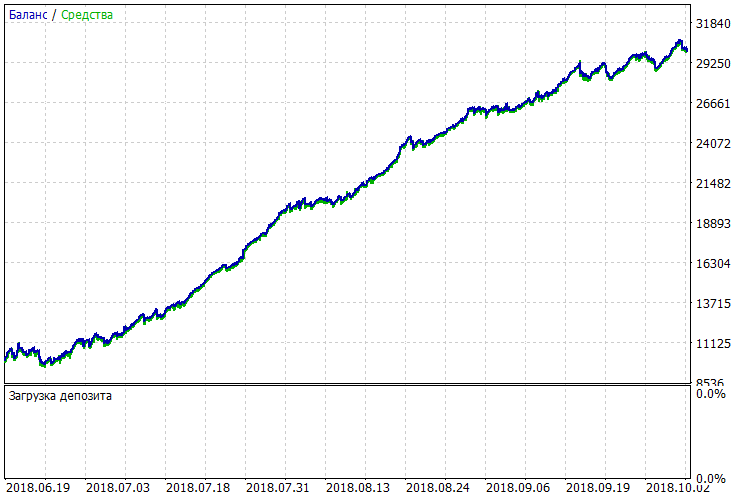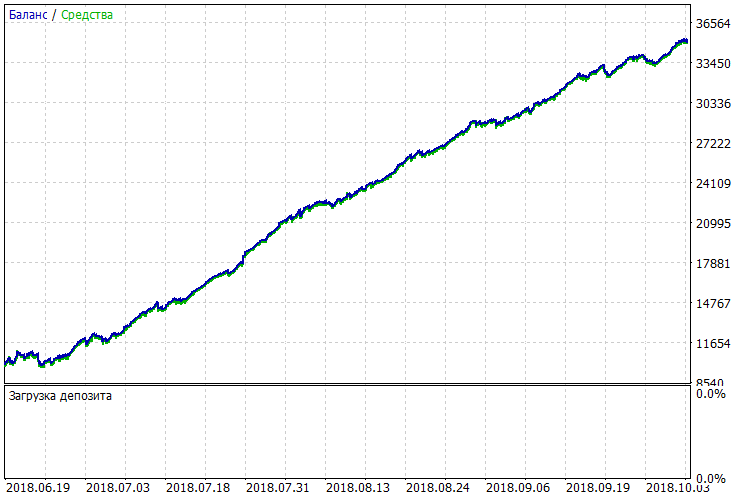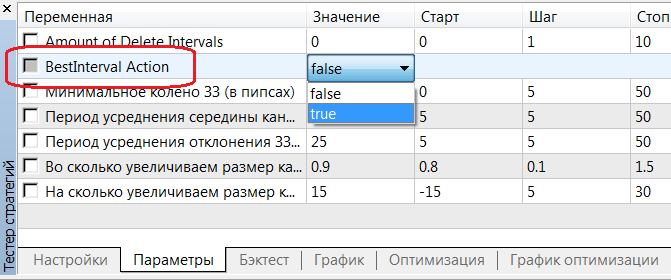Watch how to download trading robots for free

Interesting script?
So post a link to it -
let others appraise it

You liked the script? Try it in the MetaTrader 5 terminal# BestInterval - library for MetaTrader 5

Views:
1057
Rating:
votes: 24
Published:
2018.12.28 13:09
Updated:
2019.01.17 11:29

Market laws depend on the intra-day or intra-week intervals. This is why it is reasonable to limit your trading system by time.

For example, there are scalping trading systems that are good at trading at Asian and American sessions. Disabling the trading system during high volatility periods is also practiced.

Accordingly, a problem arises: How to find the best time for trading with your TS? As a rule, this interval is searched for either by intuition or by shouldering the standard Tester. Let us forget about intuition as a non-technical case. As to using the standard Tester in solving such a problem, this automatically leads to significantly increasing the number of runs and, accordingly, the Optimization and the money (Cloud). However, there will still be some points that could be improved.

It is also possible that there is a really good TS. However, its positive features are hidden, since there is no time filtere in it. After all, if it were possible to find a suitable trading interval for that TS, it would not be trashed, but would trigger additional research...

### Implementation.

This cross-platform library allows the system to find the optimal trading interval very quickly and as efficiently as possible.

Example of use with comments:

```#include <MT4Orders.mqh> // <a2>https://www.mql5.com/en/code/16006</a2>

#include <fxsaber\BestInterval\BestInterval.mqh> // Calculating the best trading interval

void OnDeinit( const int )
{
BESTINTERVAL BestInterval; // So we have created an object to calculate the best trading interval

BestInterval.Set(); // Placed trading history

const int AmountIntervals = 3; // How many worst intervals should be deleted

for (int i = 0; i < AmountIntervals; i++)
if (BestInterval.DeleteWorseInterval()) // If we have deleted something
Print(BestInterval.ToString());       // Print the trading data obtained
else
break;                                // Else, we will exit
}
```

Now, let's have a look at the real application of the library within the scalper EA.

### Scalper.

Let's launch the round-the-clock scalper in MT5 Tester. We will see the followinglook at the single pass log

```Amount of Delete Intervals = 0
00:00:00 - 23:59:59 : Profit = 20121.00, Total = 1138, PF = 1.68, Mean = 17.68
SUMMARY: 00:00:00 - 23:59:59 : Profit = 20121.00, Total = 1138, PF = 1.68, Mean = 17.68
```

here is the raw passage data - no intervals are discarded.

Now let's provide consecutively the further log entries, where the effect of deleting weak intervals would be shown gradually.

One interval is deleted:

```Amount of Delete Intervals = 1
00:00:00 - 10:08:04 : Profit = 6830.00, Total = 203, PF = 3.10, Mean = 33.65
11:05:24 - 23:59:59 : Profit = 17086.00, Total = 819, PF = 1.93, Mean = 20.86
SUMMARY: 00:00:00 - 23:59:59 : Profit = 23916.00, Total = 1022, PF = 2.10, Mean = 23.40
```

Highlighted strings are trading intervals. We can see that there is no trading from 10-11 am. The interval is deleted.

Two intervals are deleted:

```Amount of Delete Intervals = 2
00:00:00 - 10:08:04 : Profit = 6830.00, Total = 203, PF = 3.10, Mean = 33.65
11:05:24 - 12:21:06 : Profit = 2767.00, Total = 112, PF = 2.46, Mean = 24.71
13:02:59 - 23:59:59 : Profit = 15915.00, Total = 664, PF = 2.17, Mean = 23.97
SUMMARY: 00:00:00 - 23:59:59 : Profit = 25512.00, Total = 979, PF = 2.36, Mean = 26.06
```

Finally, three intervals are deleted

```Amount of Delete Intervals = 3
00:00:00 - 10:08:04 : Profit = 6830.00, Total = 203, PF = 3.10, Mean = 33.65
11:05:24 - 12:21:06 : Profit = 2767.00, Total = 112, PF = 2.46, Mean = 24.71
13:02:59 - 17:26:06 : Profit = 7476.00, Total = 348, PF = 1.98, Mean = 21.48
17:47:36 - 23:59:59 : Profit = 9640.00, Total = 288, PF = 3.31, Mean = 33.47
SUMMARY: 00:00:00 - 23:59:59 : Profit = 26713.00, Total = 951, PF = 2.57, Mean = 28.09
```

Note how the TS parameters improve with each deleted interval.

Let's apply the calculated filter to the TSWe can visually compare the two charts BEFORE and AFTER. Perhaps, someone would make an appropriate GIF animation for clarity...

As a result, the TS parameters have been improved significantly almost for free: We have spent as little efforts and time as possible.

### OnTester.

The example above was based on adding just two strings into the initial EA

```#define BESTINTERVAL_ONTESTER // Optimization criterion is the profit of the best interval.
#include <fxsaber\BestInterval\BestInterval.mqh> // Calculating the best trading interval
```

The highlighted string allows you not to write anything else in the EA. At the same time, the custom optimization criterion is also specified, allowing the optimizer not to look for good intervals in the old-fashioned way. The criterion immediately returns the TS result on a good interval calculated and show the TS potential much better than when using standard Optimization.

To apply the found interval upon calculating thereof, set BestInteval Action = true in its inputs.### Summary.

All TSes should be broadly checked, including the best trading interval calculation. Therefore, this tool is a must-have.

It is reasonable to connect to all current, past, and future TSes (using the two strings above) the search for an optimal trading interval. This implementation facilitates considerably creating a profitable TS or improving the robustness of an existing one

### Features.

• It is recommended to enable/disable trading on the intervals detected, using the virtual trading environment.
• The library algorithm is very fast. It can be easily implemented in third-party trading analyzers for the same purpose.
• This is my own idea. Perhaps there are similar solutions.
• I have not got around yet to implement intra-week intervals. Maybe later...
• The library is a cross-platform one. It is relevant for both MT4 and MT5.
• The best interval is calculated and then applied in the Tester without modifying the original EA source code.

### P.S.

Please share your experience of applying the library BEFORE and AFTER (as described above) as screenshots and appropriate MT4/5 single pass log segments.

Translated from Russian by MetaQuotes Software Corp.
Original code: https://www.mql5.com/ru/code/22710HLCrossSigForWPR_HTF

Indicator HLCrossSigForWPR with the option of selecting its timeframe in its input parametersRJTX_Matches_Smoothed_Alert

Indicator RJTX_Matches_Smoothed featuring alerts, emails and push notifications for smartphonesPrice_Momentum_Oscillator

Indicator Price Momentum OscillatorExtreme EA

Indicators iCCI (Commodity Channel Index, CCI) and two iMAs (Moving Average, MA) are used.